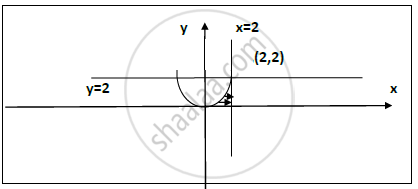Advertisement Remove all ads

# Change the Order of Integration and Evaluate ∫ 2 0 ∫ 2 √ 2 Y X 2 √ X 4 − 4 Y 2 D X D Y - Applied Mathematics 2

Sum

Change the order of Integration and evaluate int_0^2int_sqrt(2y)^2 x^2/(sqrtx^4-4y^2)dxdy

Advertisement Remove all ads

#### Solution

Let I = int_0^2int_sqrt(2y)^2 x^2/(sqrtx^4-4y^2)dxdy
Region of integration : sqrt(2y)<=x<=2

0<=y<=2

Curves : (i) x = 2 , y = 2 , y = 0 are lines.
(ii) x=sqrt(2y)=>x^2=2y

Parabola with vertex (0,0) opening in upward direction.After changing the order of integration:

0<=y<=x^2/2

0<=x<=2

therefore "I"=int_0^2int_0^(x^2/2)x^2/sqrt(x^4-4y^2)dydx

=1/2int_0^2int_0^(x^2/2)x^2/sqrt(x^4/4-y^2)dydx

=1/2int_0^2x^2[sin^(-1)(y/(x^2/2))]_0^(x^2/2)dy

therefore  "I"=1/2int_0^2x^2pi/2dx

=pi/4[x^3/3]_0^2

therefore "I"=(2x)/3

Concept: Change the Order of Integration
Is there an error in this question or solution?
Advertisement Remove all ads

#### APPEARS IN

Advertisement Remove all ads
Advertisement Remove all ads
Share
Notifications

View all notifications

Forgot password?